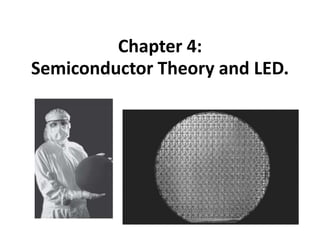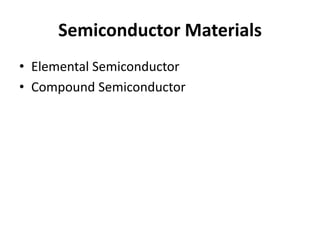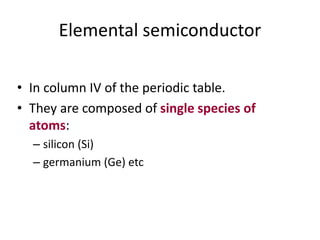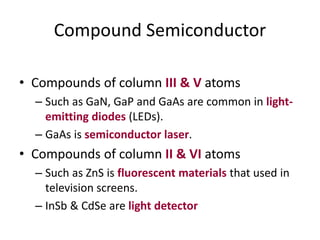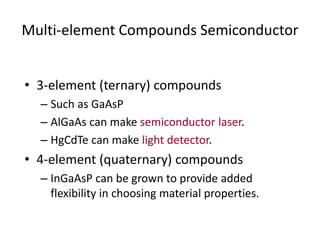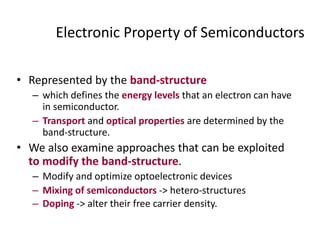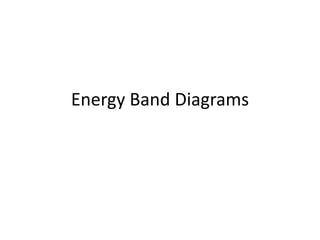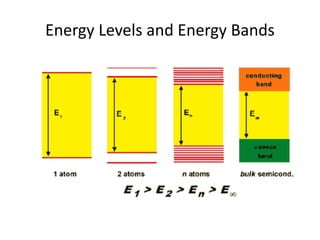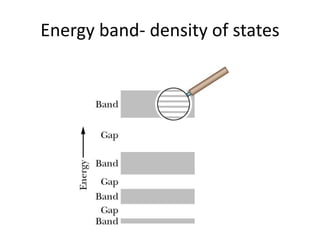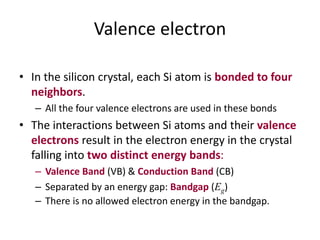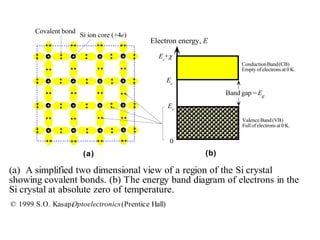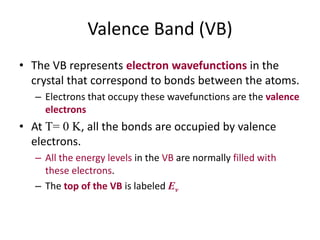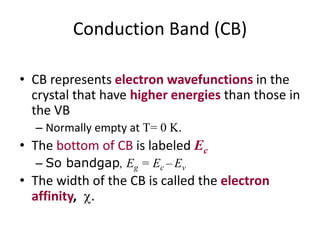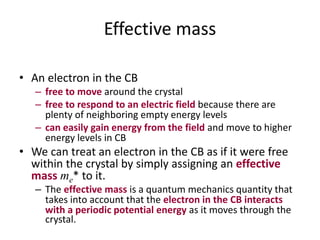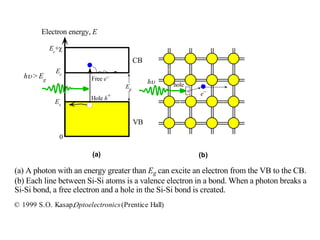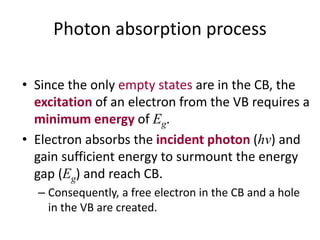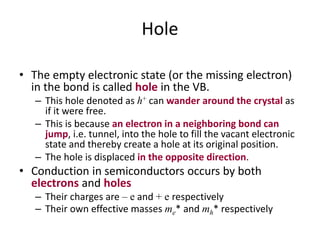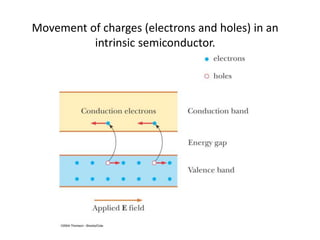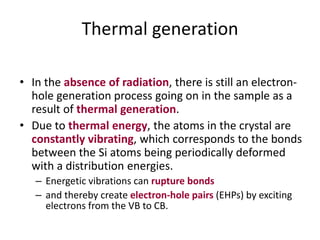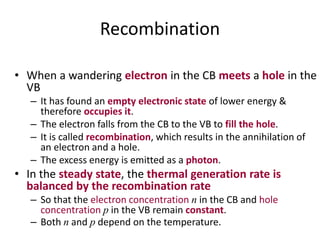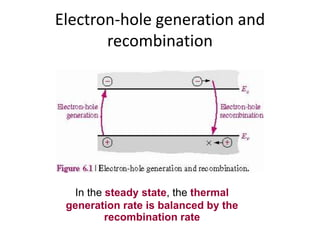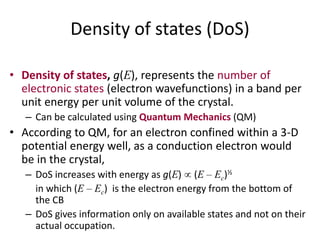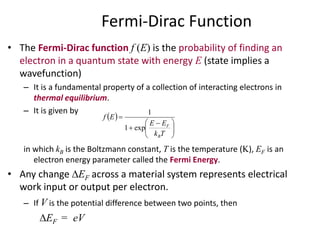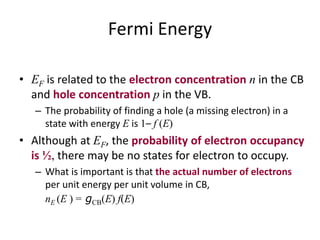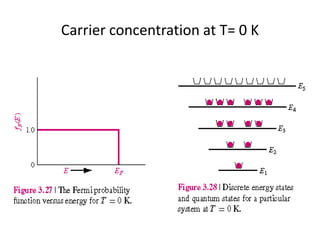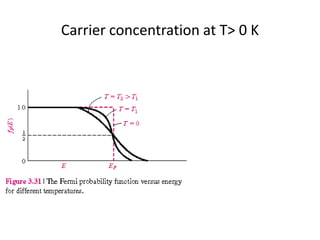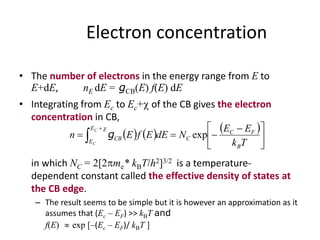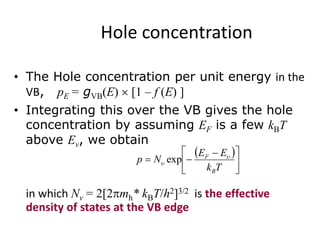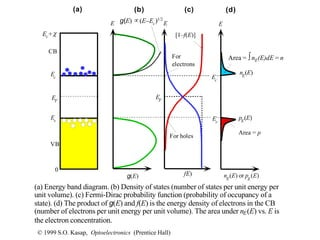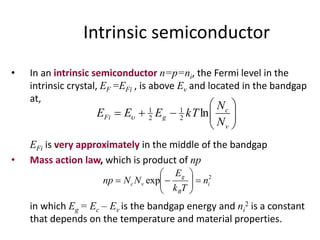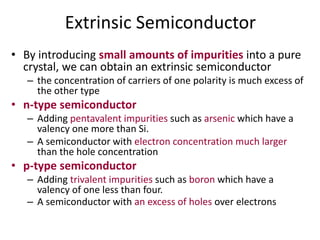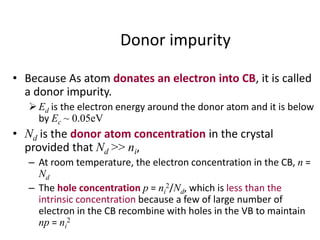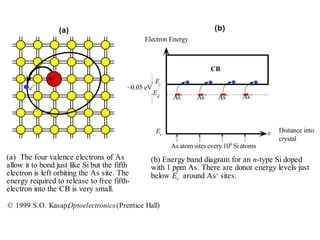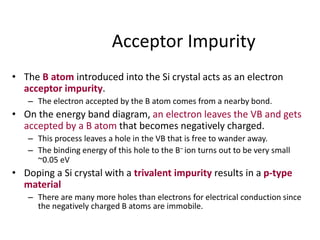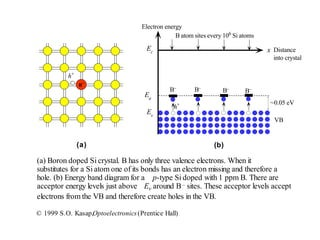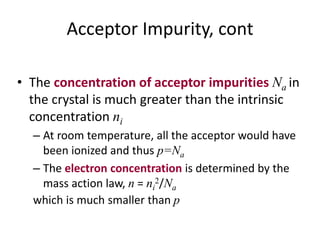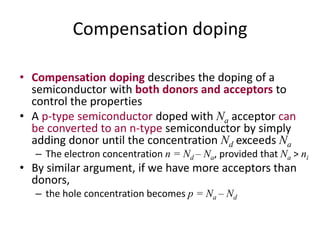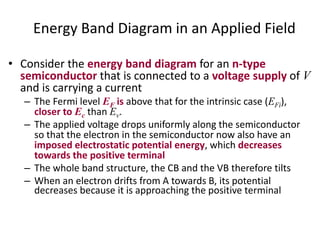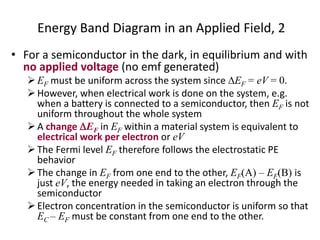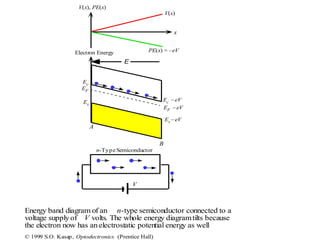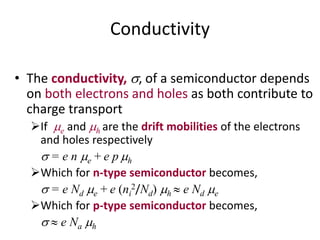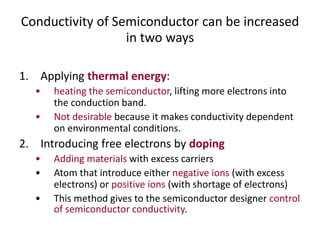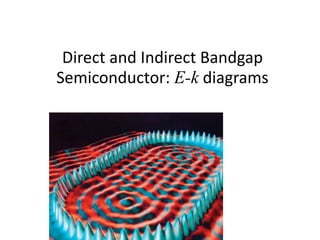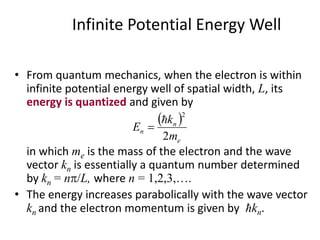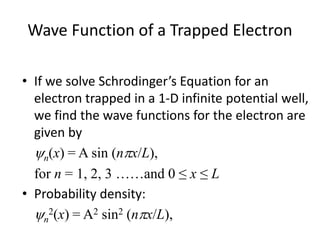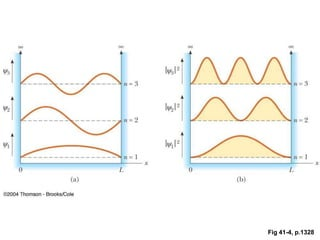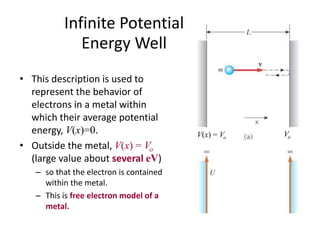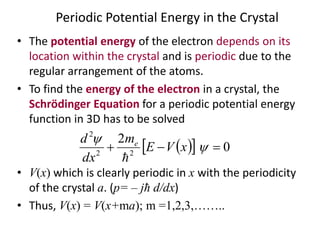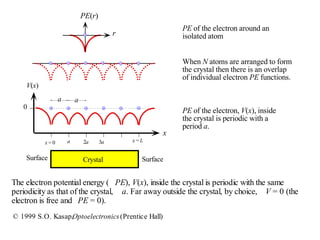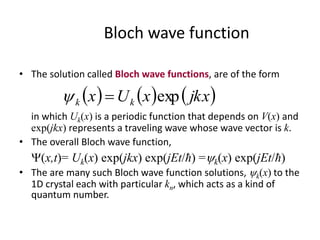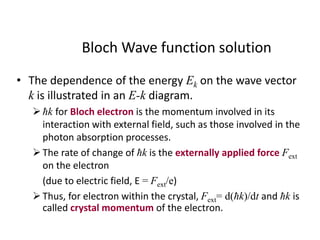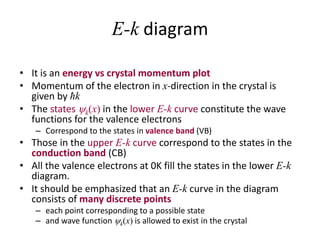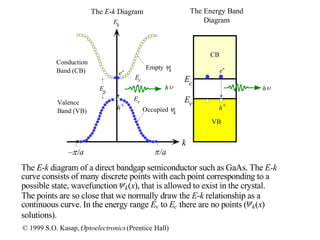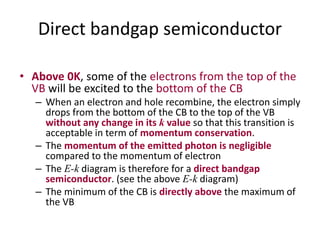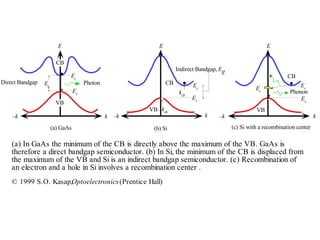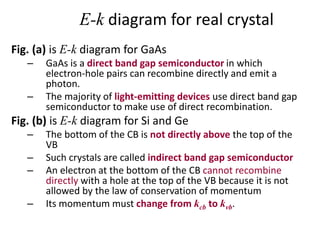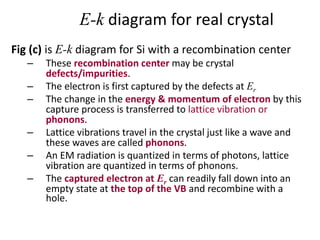1 de 58

### Chapter 4a

1. Chapter 4: Semiconductor Theory and LED.
2. Semiconductor Materials • Elemental Semiconductor • Compound Semiconductor
3. Elemental semiconductor • In column IV of the periodic table. • They are composed of single species of atoms: – silicon (Si) – germanium (Ge) etc
4. Compound Semiconductor • Compounds of column III & V atoms – Such as GaN, GaP and GaAs are common in light- emitting diodes (LEDs). – GaAs is semiconductor laser. • Compounds of column II & VI atoms – Such as ZnS is fluorescent materials that used in television screens. – InSb & CdSe are light detector
5. Multi-element Compounds Semiconductor • 3-element (ternary) compounds – Such as GaAsP – AlGaAs can make semiconductor laser. – HgCdTe can make light detector. • 4-element (quaternary) compounds – InGaAsP can be grown to provide added flexibility in choosing material properties.
6. Electronic Property of Semiconductors • Represented by the band-structure – which defines the energy levels that an electron can have in semiconductor. – Transport and optical properties are determined by the band-structure. • We also examine approaches that can be exploited to modify the band-structure. – Modify and optimize optoelectronic devices – Mixing of semiconductors -> hetero-structures – Doping -> alter their free carrier density.
7. Energy Band Diagrams
8. Energy Levels and Energy Bands
9. Energy band- density of states
10. Valence electron • In the silicon crystal, each Si atom is bonded to four neighbors. – All the four valence electrons are used in these bonds • The interactions between Si atoms and their valence electrons result in the electron energy in the crystal falling into two distinct energy bands: – Valence Band (VB) & Conduction Band (CB) – Separated by an energy gap: Bandgap (Eg) – There is no allowed electron energy in the bandgap.
11. Electron energy, E ConductionBand(CB) Empty of electrons at 0 K. ValenceBand(VB) Full of electrons at 0 K. Ec Ev 0 Ec + (b) Band gap = Eg (a) Covalent bond Si ion core (+4e) (a) A simplified two dimensional view of a region of the Si crystal showing covalent bonds. (b) The energy band diagram of electrons in the Si crystal at absolute zero of temperature. © 1999 S.O. Kasap,Optoelectronics(Prentice Hall)
12. Valence Band (VB) • The VB represents electron wavefunctions in the crystal that correspond to bonds between the atoms. – Electrons that occupy these wavefunctions are the valence electrons • At T= 0 K, all the bonds are occupied by valence electrons. – All the energy levels in the VB are normally filled with these electrons. – The top of the VB is labeled Ev
13. Conduction Band (CB) • CB represents electron wavefunctions in the crystal that have higher energies than those in the VB – Normally empty at T= 0 K. • The bottom of CB is labeled Ec – So bandgap, Eg = Ec – Ev • The width of the CB is called the electron affinity, .
14. Effective mass • An electron in the CB – free to move around the crystal – free to respond to an electric field because there are plenty of neighboring empty energy levels – can easily gain energy from the field and move to higher energy levels in CB • We can treat an electron in the CB as if it were free within the crystal by simply assigning an effective mass me* to it. – The effective mass is a quantum mechanics quantity that takes into account that the electron in the CB interacts with a periodic potential energy as it moves through the crystal.
15. e– hole CB VB Ec Ev 0 Ec + Eg Free e–h > Eg Hole h+ Electron energy, E (a) A photon with an energy greater than Eg can excite an electron from the VB to the CB. (b) Each line between Si-Si atoms is a valence electron in a bond. When a photon breaks a Si-Si bond, a free electron and a hole in the Si-Si bond is created. h (a) (b) © 1999 S.O. Kasap,Optoelectronics(Prentice Hall)
16. Photon absorption process • Since the only empty states are in the CB, the excitation of an electron from the VB requires a minimum energy of Eg. • Electron absorbs the incident photon (hv) and gain sufficient energy to surmount the energy gap (Eg) and reach CB. – Consequently, a free electron in the CB and a hole in the VB are created.
17. Hole • The empty electronic state (or the missing electron) in the bond is called hole in the VB. – This hole denoted as h+ can wander around the crystal as if it were free. – This is because an electron in a neighboring bond can jump, i.e. tunnel, into the hole to fill the vacant electronic state and thereby create a hole at its original position. – The hole is displaced in the opposite direction. • Conduction in semiconductors occurs by both electrons and holes – Their charges are – e and + e respectively – Their own effective masses me* and mh* respectively
18. Movement of charges (electrons and holes) in an intrinsic semiconductor.
19. Thermal generation • In the absence of radiation, there is still an electron- hole generation process going on in the sample as a result of thermal generation. • Due to thermal energy, the atoms in the crystal are constantly vibrating, which corresponds to the bonds between the Si atoms being periodically deformed with a distribution energies. – Energetic vibrations can rupture bonds – and thereby create electron-hole pairs (EHPs) by exciting electrons from the VB to CB.
20. Recombination • When a wandering electron in the CB meets a hole in the VB – It has found an empty electronic state of lower energy & therefore occupies it. – The electron falls from the CB to the VB to fill the hole. – It is called recombination, which results in the annihilation of an electron and a hole. – The excess energy is emitted as a photon. • In the steady state, the thermal generation rate is balanced by the recombination rate – So that the electron concentration n in the CB and hole concentration p in the VB remain constant. – Both n and p depend on the temperature.
21. Electron-hole generation and recombination In the steady state, the thermal generation rate is balanced by the recombination rate
22. Density of states (DoS) • Density of states, g(E), represents the number of electronic states (electron wavefunctions) in a band per unit energy per unit volume of the crystal. – Can be calculated using Quantum Mechanics (QM) • According to QM, for an electron confined within a 3-D potential energy well, as a conduction electron would be in the crystal, – DoS increases with energy as g(E) (E – Ec)½ in which (E – Ec) is the electron energy from the bottom of the CB – DoS gives information only on available states and not on their actual occupation.
23. Fermi-Dirac Function • The Fermi-Dirac function f (E) is the probability of finding an electron in a quantum state with energy E (state implies a wavefunction) – It is a fundamental property of a collection of interacting electrons in thermal equilibrium. – It is given by in which kB is the Boltzmann constant, T is the temperature (K), EF is an electron energy parameter called the Fermi Energy. • Any change EF across a material system represents electrical work input or output per electron. – If V is the potential difference between two points, then EF = eV            Tk EE Ef B F exp1 1
24. Fermi Energy • EF is related to the electron concentration n in the CB and hole concentration p in the VB. – The probability of finding a hole (a missing electron) in a state with energy E is 1– f (E) • Although at EF, the probability of electron occupancy is ½, there may be no states for electron to occupy. – What is important is that the actual number of electrons per unit energy per unit volume in CB, nE (E ) = gCB(E) f(E)
25. Carrier concentration at T= 0 K
26. Carrier concentration at T> 0 K
27. Electron concentration • The number of electrons in the energy range from E to E+dE, nE dE = gCB(E) f(E) dE • Integrating from Ec to Ec+ of the CB gives the electron concentration in CB, in which NC = 2[2me* kBT/h2]3/2 is a temperature- dependent constant called the effective density of states at the CB edge. – The result seems to be simple but it is however an approximation as it assumes that (Ec – EF) >> kBT and f(E)  exp [–(Ec – EF)/ kBT ]                 Tk EE NdEEfEn B FC C E E CB C C exp  g
28. Hole concentration • The Hole concentration per unit energy in the VB, pE = gVB(E) [1 – f (E) ] • Integrating this over the VB gives the hole concentration by assuming EF is a few kBT above Ev, we obtain in which Nv = 2[2mh* kBT/h2]3/2 is the effective density of states at the VB edge           Tk EE Np B F   exp
29. E g(E) fE) EF nE (E) or pE (E) E E For electrons For holes [1–f(E)] nE (E) pE (E) Area = p Area = ∫nE(E)dE = n Ec Ev Ev Ec 0 Ec+ EF VB CB (a) (b) (c) (d) g(E) (E–Ec)1/2 (a) Energy band diagram. (b) Density of states (number of states per unit energy per unit volume). (c) Fermi-Dirac probability function (probability of occupancy of a state). (d) The product of g(E) and f(E) is the energy density of electrons in the CB (number of electrons per unit energy per unit volume). The area under nE(E) vs. E is the electron concentration. © 1999 S.O. Kasap, Optoelectronics (Prentice Hall)
30. Intrinsic semiconductor • In an intrinsic semiconductor n=p=ni, the Fermi level in the intrinsic crystal, EF =EFi , is above Ev and located in the bandgap at, EFi is very approximately in the middle of the bandgap • Mass action law, which is product of np in which Eg = Ec – Ev is the bandgap energy and ni 2 is a constant that depends on the temperature and material properties.        v c gFi N N kTEEE ln2 1 2 1  2 exp i B g vc n Tk E NNnp       
31. Extrinsic Semiconductor • By introducing small amounts of impurities into a pure crystal, we can obtain an extrinsic semiconductor – the concentration of carriers of one polarity is much excess of the other type • n-type semiconductor – Adding pentavalent impurities such as arsenic which have a valency one more than Si. – A semiconductor with electron concentration much larger than the hole concentration • p-type semiconductor – Adding trivalent impurities such as boron which have a valency of one less than four. – A semiconductor with an excess of holes over electrons
32. Donor impurity • Because As atom donates an electron into CB, it is called a donor impurity. Ed is the electron energy around the donor atom and it is below by Ec ~ 0.05eV • Nd is the donor atom concentration in the crystal provided that Nd >> ni, – At room temperature, the electron concentration in the CB, n = Nd – The hole concentration p = ni 2/Nd, which is less than the intrinsic concentration because a few of large number of electron in the CB recombine with holes in the VB to maintain np = ni 2
33. e– (a) As+ x As+ As+ As+ As+ Ec Ed CB Ev ~0.05 eV As atom sites every 106 Si atoms Distance into crystal (b) Electron Energy (a) The four valence electrons of As allow it to bond just like Si but the fifth electron is left orbiting the As site. The energy required to release to free fifth- electron into the CB is very small. (b) Energy band diagram for an n-type Si doped with 1 ppm As. There are donor energy levels just below Ec around As+ sites. © 1999 S.O. Kasap,Optoelectronics(Prentice Hall)
34. Acceptor Impurity • The B atom introduced into the Si crystal acts as an electron acceptor impurity. – The electron accepted by the B atom comes from a nearby bond. • On the energy band diagram, an electron leaves the VB and gets accepted by a B atom that becomes negatively charged. – This process leaves a hole in the VB that is free to wander away. – The binding energy of this hole to the B– ion turns out to be very small ~0.05 eV • Doping a Si crystal with a trivalent impurity results in a p-type material – There are many more holes than electrons for electrical conduction since the negatively charged B atoms are immobile.
35. B– h+ (a) x B– Ev Ea B atom sites every 106 Si atoms Distance into crystal ~0.05 eV B– B– B– h+ VB Ec Electron energy (b) (a) Boron doped Si crystal. B has only three valence electrons. When it substitutes for a Si atom one of its bonds has an electron missing and therefore a hole. (b) Energy band diagram for a p-type Si doped with 1 ppm B. There are acceptor energy levels just above Ev around B– sites. These acceptor levels accept electrons from the VB and therefore create holes in the VB. © 1999 S.O. Kasap,Optoelectronics(Prentice Hall)
36. Acceptor Impurity, cont • The concentration of acceptor impurities Na in the crystal is much greater than the intrinsic concentration ni – At room temperature, all the acceptor would have been ionized and thus p=Na – The electron concentration is determined by the mass action law, n = ni 2/Na which is much smaller than p
37. Compensation doping • Compensation doping describes the doping of a semiconductor with both donors and acceptors to control the properties • A p-type semiconductor doped with Na acceptor can be converted to an n-type semiconductor by simply adding donor until the concentration Nd exceeds Na – The electron concentration n = Nd – Na, provided that Na > ni • By similar argument, if we have more acceptors than donors, – the hole concentration becomes p = Na – Nd
38. Energy Band Diagram in an Applied Field • Consider the energy band diagram for an n-type semiconductor that is connected to a voltage supply of V and is carrying a current – The Fermi level EF is above that for the intrinsic case (EFi), closer to Ec than Ev. – The applied voltage drops uniformly along the semiconductor so that the electron in the semiconductor now also have an imposed electrostatic potential energy, which decreases towards the positive terminal – The whole band structure, the CB and the VB therefore tilts – When an electron drifts from A towards B, its potential decreases because it is approaching the positive terminal
39. Energy Band Diagram in an Applied Field, 2 • For a semiconductor in the dark, in equilibrium and with no applied voltage (no emf generated) EF must be uniform across the system since EF = eV = 0. However, when electrical work is done on the system, e.g. when a battery is connected to a semiconductor, then EF is not uniform throughout the whole system A change EF in EF within a material system is equivalent to electrical work per electron or eV The Fermi level EF therefore follows the electrostatic PE behavior The change in EF from one end to the other, EF(A) – EF(B) is just eV, the energy needed in taking an electron through the semiconductor Electron concentration in the semiconductor is uniform so that EC – EF must be constant from one end to the other.
40. V n-Type Semiconductor Ec EF  eV A B V(x), PE(x) x PE(x) = –eV Energy band diagram of an n-type semiconductor connected to a voltage supplyof V volts. The whole energy diagramtilts because the electron now has anelectrostatic potential energy as well E Electron Energy Ec  eV Ev eV V(x) EF Ev © 1999 S.O. Kasap, Optoelectronics (Prentice Hall)
41. Conductivity • The conductivity, , of a semiconductor depends on both electrons and holes as both contribute to charge transport If e and h are the drift mobilities of the electrons and holes respectively  = e n e + e p h Which for n-type semiconductor becomes,  = e Nd e + e (ni 2/Nd) h  e Nd e Which for p-type semiconductor becomes,   e Na h
42. Conductivity of Semiconductor can be increased in two ways 1. Applying thermal energy: • heating the semiconductor, lifting more electrons into the conduction band. • Not desirable because it makes conductivity dependent on environmental conditions. 2. Introducing free electrons by doping • Adding materials with excess carriers • Atom that introduce either negative ions (with excess electrons) or positive ions (with shortage of electrons) • This method gives to the semiconductor designer control of semiconductor conductivity.
43. Direct and Indirect Bandgap Semiconductor: E-k diagrams
44. Infinite Potential Energy Well • From quantum mechanics, when the electron is within infinite potential energy well of spatial width, L, its energy is quantized and given by in which me is the mass of the electron and the wave vector kn is essentially a quantum number determined by kn = n/L, where n = 1,2,3,…. • The energy increases parabolically with the wave vector kn and the electron momentum is given by ħkn.   e n n m k E 2 2  
45. Wave Function of a Trapped Electron • If we solve Schrodinger’s Equation for an electron trapped in a 1-D infinite potential well, we find the wave functions for the electron are given by n(x) = A sin (nx/L), for n = 1, 2, 3 ……and 0 ≤ x ≤ L • Probability density: n 2(x) = A2 sin2 (nx/L),
46. Fig 41-4, p.1328
47. Infinite Potential Energy Well • This description is used to represent the behavior of electrons in a metal within which their average potential energy, V(x)=0. • Outside the metal, V(x) = Vo (large value about several eV) – so that the electron is contained within the metal. – This is free electron model of a metal. V(x) = Vo Vo
48. Periodic Potential Energy in the Crystal • The potential energy of the electron depends on its location within the crystal and is periodic due to the regular arrangement of the atoms. • To find the energy of the electron in a crystal, the Schrödinger Equation for a periodic potential energy function in 3D has to be solved • V(x) which is clearly periodic in x with the periodicity of the crystal a. (p= – jħ d/dx) • Thus, V(x) = V(x+ma); m =1,2,3,……..    0 2 22 2    xVE m dx d e 
49. r PE(r) PE of the electron around an isolated atom When N atoms are arranged to form the crystal then there is an overlap of individual electron PE functions. x V(x) x = Lx = 0 a 2a 3a 0 aa Surface SurfaceCrystal PE of the electron, V(x), inside the crystal is periodic with a period a. The electron potential energy ( PE), V(x), inside the crystal is periodic with the same periodicity as that of the crystal, a. Far away outside the crystal, by choice, V = 0 (the electron is free and PE = 0). © 1999 S.O. Kasap,Optoelectronics(Prentice Hall)
50. Bloch wave function • The solution called Bloch wave functions, are of the form in which Uk(x) is a periodic function that depends on V(x) and exp(jkx) represents a traveling wave whose wave vector is k. • The overall Bloch wave function, (x,t)= Uk(x) exp(jkx) exp(jEt/ħ) =k(x) exp(jEt/ħ) • The are many such Bloch wave function solutions, k(x) to the 1D crystal each with particular kn, which acts as a kind of quantum number.      jkxxUx kk exp
51. Bloch Wave function solution • The dependence of the energy Ek on the wave vector k is illustrated in an E-k diagram. ħk for Bloch electron is the momentum involved in its interaction with external field, such as those involved in the photon absorption processes. The rate of change of ħk is the externally applied force Fext on the electron (due to electric field, E = Fext/e) Thus, for electron within the crystal, Fext= d(ħk)/dt and ħk is called crystal momentum of the electron.
52. E-k diagram • It is an energy vs crystal momentum plot • Momentum of the electron in x-direction in the crystal is given by ħk • The states k(x) in the lower E-k curve constitute the wave functions for the valence electrons – Correspond to the states in valence band (VB) • Those in the upper E-k curve correspond to the states in the conduction band (CB) • All the valence electrons at 0K fill the states in the lower E-k diagram. • It should be emphasized that an E-k curve in the diagram consists of many discrete points – each point corresponding to a possible state – and wave function k(x) is allowed to exist in the crystal
53. Ek k /a–/a Ec Ev Conduction Band (CB) Ec Ev CB The E-k Diagram The Energy Band Diagram Empty k Occupied k h+ e- Eg e- h+ h VB h Valence Band (VB) The E-k diagram of a direct bandgap semiconductor such as GaAs. The E-k curve consists of many discrete points with each point corresponding to a possible state, wavefunctionk(x), that is allowed to exist in the crystal. The points are so close that we normally draw the E-k relationship as a continuous curve. In the energy range Ev to Ec there are no points (k(x) solutions). © 1999 S.O. Kasap, Optoelectronics(Prentice Hall)
54. Direct bandgap semiconductor • Above 0K, some of the electrons from the top of the VB will be excited to the bottom of the CB – When an electron and hole recombine, the electron simply drops from the bottom of the CB to the top of the VB without any change in its k value so that this transition is acceptable in term of momentum conservation. – The momentum of the emitted photon is negligible compared to the momentum of electron – The E-k diagram is therefore for a direct bandgap semiconductor. (see the above E-k diagram) – The minimum of the CB is directly above the maximum of the VB
55. E CB k–k Direct Bandgap (a) GaAs E CB VB Indirect Bandgap, Eg k–k kcb (b) Si E k–k Phonon (c) Si with a recombination center Eg Ec Ev Ec Ev kvb VB CB Er Ec Ev Photon VB (a) In GaAs the minimum of the CB is directly above the maximum of the VB. GaAs is therefore a direct bandgap semiconductor. (b) In Si, the minimum of the CB is displaced from the maximum of the VB and Si is an indirect bandgap semiconductor. (c) Recombination of an electron and a hole in Si involves a recombination center . © 1999 S.O. Kasap,Optoelectronics(Prentice Hall)
56. E-k diagram for real crystal Fig. (a) is E-k diagram for GaAs – GaAs is a direct band gap semiconductor in which electron-hole pairs can recombine directly and emit a photon. – The majority of light-emitting devices use direct band gap semiconductor to make use of direct recombination. Fig. (b) is E-k diagram for Si and Ge – The bottom of the CB is not directly above the top of the VB – Such crystals are called indirect band gap semiconductor – An electron at the bottom of the CB cannot recombine directly with a hole at the top of the VB because it is not allowed by the law of conservation of momentum – Its momentum must change from kcb to kvb.
57. E CB k–k Direct Bandgap (a) GaAs E CB VB Indirect Bandgap, Eg k–k kcb (b) Si E k–k Phonon (c) Si with a recombination center Eg Ec Ev Ec Ev kvb VB CB Er Ec Ev Photon VB (a) In GaAs the minimum of the CB is directly above the maximum of the VB. GaAs is therefore a direct bandgap semiconductor. (b) In Si, the minimum of the CB is displaced from the maximum of the VB and Si is an indirect bandgap semiconductor. (c) Recombination of an electron and a hole in Si involves a recombination center . © 1999 S.O. Kasap,Optoelectronics(Prentice Hall)
58. E-k diagram for real crystal Fig (c) is E-k diagram for Si with a recombination center – These recombination center may be crystal defects/impurities. – The electron is first captured by the defects at Er – The change in the energy & momentum of electron by this capture process is transferred to lattice vibration or phonons. – Lattice vibrations travel in the crystal just like a wave and these waves are called phonons. – An EM radiation is quantized in terms of photons, lattice vibration are quantized in terms of phonons. – The captured electron at Er can readily fall down into an empty state at the top of the VB and recombine with a hole.